American National Standard Preferred Hole Basis Metric Clearance Fits
ANSI B4.2-1978 (R2004)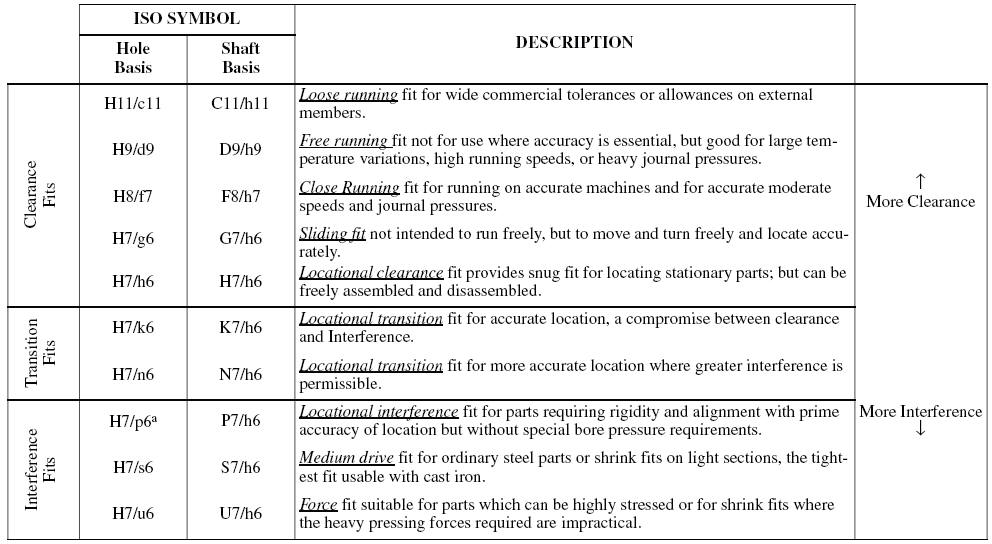a Transition fit for basic sizes in range from 0 through 3 mm.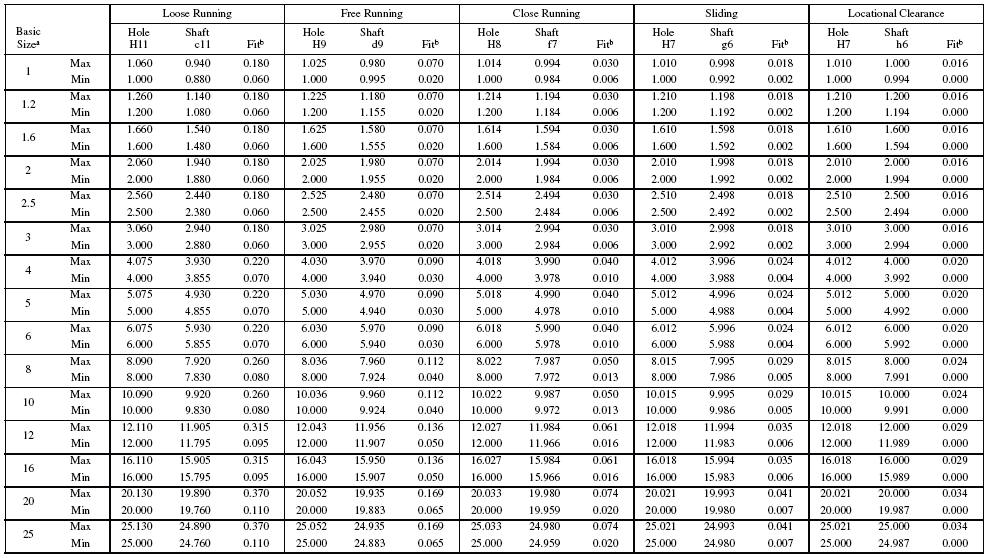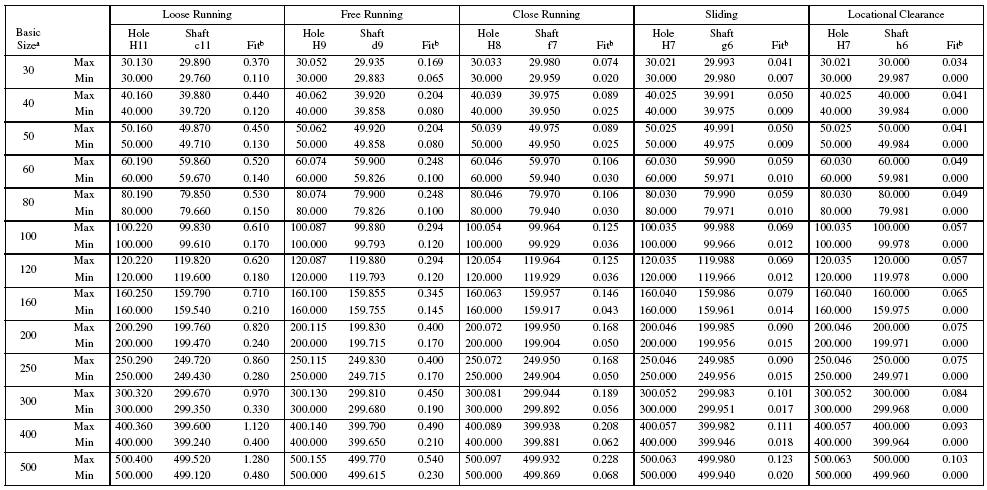a The sizes shown are first-choice basic sizes. Preferred fits for other sizes can be calculated from data given in ANSI B4.2-1978 (R2004).
b All fits shown in this table have clearance.
All dimensions are in millimeters.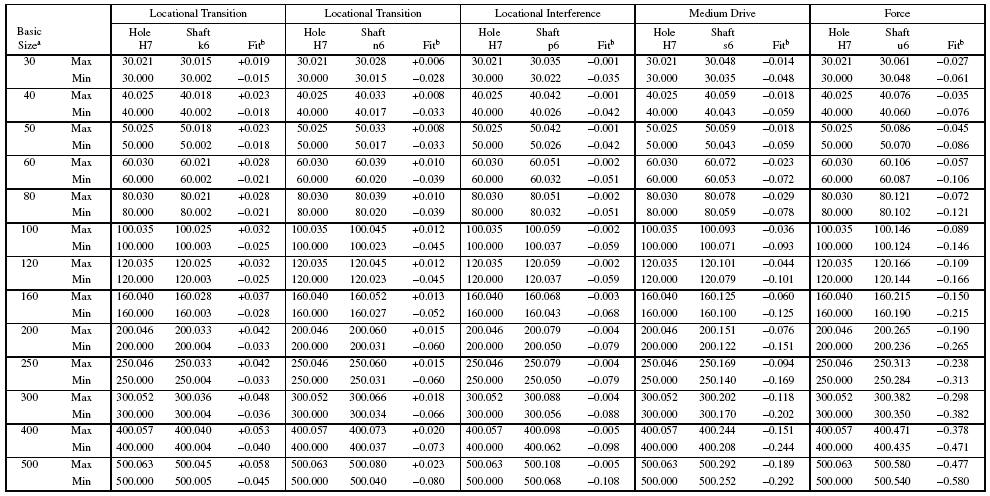a The sizes shown are first-choice basic sizes. Preferred fits for other sizes can be calculated from data given in ANSI B4.2-1978 (R2004).
All dimensions are in millimeters.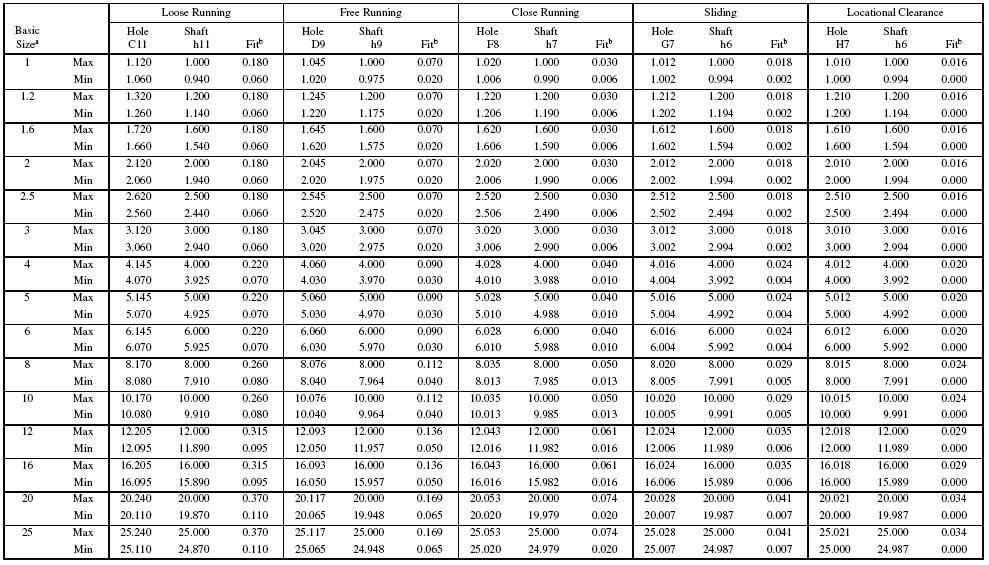a The sizes shown are first-choice basic sizes. Preferred fits for other sizes can be calculated from data given in ANSI B4.2-1978 (R2004).
All dimensions are in millimeters.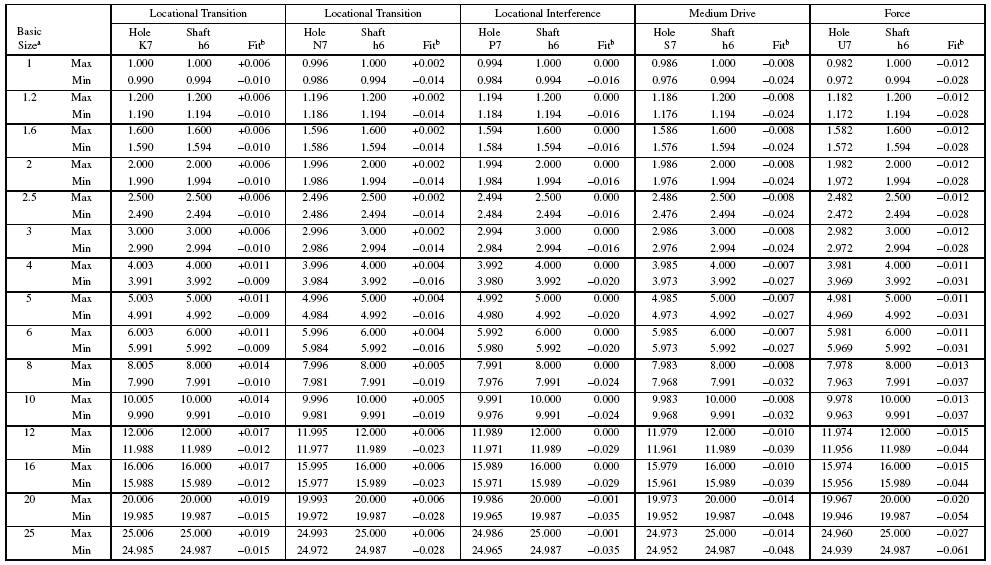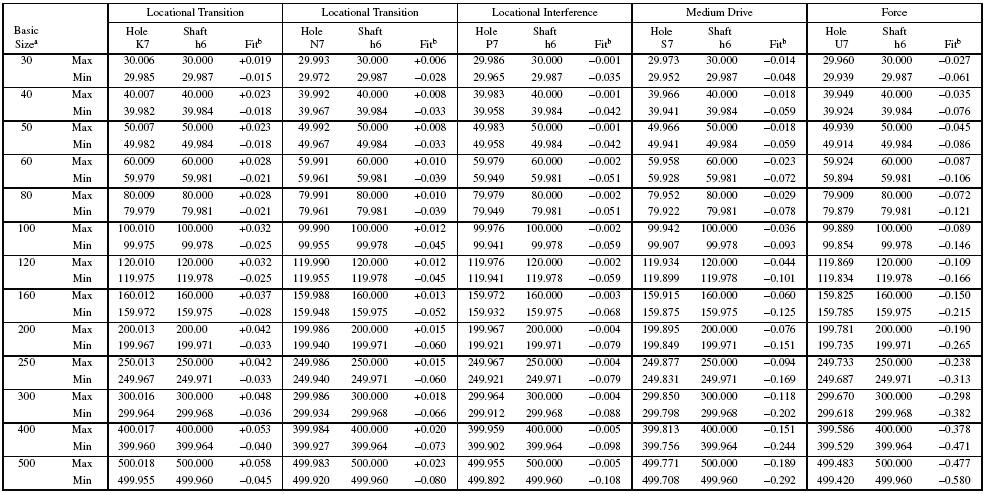a The sizes shown are first-choice basic sizes. Preferred fits for other sizes can be calculated from data given in ANSI B4.2-1978 (R2004).